# User:Tohline/H BookTiledMenu

(Difference between revisions)
Jump to: navigation, search
 Revision as of 18:05, 3 August 2019 (view source)Tohline (Talk | contribs) (→Axisymmetric Equilibrium Structures)← Older edit Revision as of 21:18, 11 August 2019 (view source)Tohline (Talk | contribs) (→Sheroidal & Spheroidal-Like)Newer edit → Line 443: Line 443: * [https://ui.adsabs.harvard.edu/abs/1977ApJ...213..497H/abstract C. Hunter (1977)], ApJ, 213, 497:  ''On Secular Stability, Secular Instability, and Points of Bifurcation of Rotating Gaseous Masses'' * [https://ui.adsabs.harvard.edu/abs/1977ApJ...213..497H/abstract C. Hunter (1977)], ApJ, 213, 497:  ''On Secular Stability, Secular Instability, and Points of Bifurcation of Rotating Gaseous Masses'' * [https://ui.adsabs.harvard.edu/abs/1985ApJ...294..474I/abstract J. N. Imamura, J. L. Friedman & R. H. Durisen (1985)], ApJ, 294, 474:  ''Secular stability limits for rotating polytropic stars'' * [https://ui.adsabs.harvard.edu/abs/1985ApJ...294..474I/abstract J. N. Imamura, J. L. Friedman & R. H. Durisen (1985)], ApJ, 294, 474:  ''Secular stability limits for rotating polytropic stars'' +
+ The equilibrium models are calculated using the polytrope version (Bodenheimer & Ostriker 1973) of the Ostriker and Mark (1968) self-consistent field (SCF) code … the equilibrium models rotate on cylinders and are completely specified by $~n$, the total angular momentum, and the specific angular momentum distribution $~j(m_\varpi)$.  Here $~m_\varpi$ is the mass contained within a cylinder of radius $~\varpi$ centered on the rotation axis.  The angular momentum distribution is prescribed in several ways:  (1) imposing strict uniform rotation;  (2) using the same $~j(m_\varpi)$ as that of a uniformly rotating spherical polybrope of index $~n^'$ (see Bodenheimer and Ostriker 1973); and (3) using $~j(m_\varpi) \propto m_\varpi$, which we refer to as $~n^' = L$, $~L$ for "linear." +
+ * [https://ui.adsabs.harvard.edu/abs/1990ApJ...355..226I/abstract J. R. Ipser & L. Lindblom (1990)], ApJ, 355, 226:  ''The Oscillations of Rapidly Rotating Newtonian Stellar Models'' * [https://ui.adsabs.harvard.edu/abs/1990ApJ...355..226I/abstract J. R. Ipser & L. Lindblom (1990)], ApJ, 355, 226:  ''The Oscillations of Rapidly Rotating Newtonian Stellar Models'' * [https://ui.adsabs.harvard.edu/abs/1991ApJ...373..213I/abstract J. R. Ipser & L. Lindblom (1991)], ApJ, 373, 213:  ''The Oscillations of Rapidly Rotating Newtonian Stellar Models.  II. Dissipative Effects'' * [https://ui.adsabs.harvard.edu/abs/1991ApJ...373..213I/abstract J. R. Ipser & L. Lindblom (1991)], ApJ, 373, 213:  ''The Oscillations of Rapidly Rotating Newtonian Stellar Models.  II. Dissipative Effects''

# Tiled Menu

 |   Tiled Menu   |   Tables of Content   |  Banner Video   |  Tohline Home Page   |

## Spherically Symmetric Configurations

(Initially) Spherically Symmetric Configurations

### Equilibrium Structures

1D STRUCTURE

Hydrostatic
Balance
Equation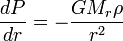$~\frac{dP}{dr} = - \frac{GM_r \rho}{r^2}$
Solution
Strategies

Isothermal
Sphere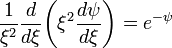$~\frac{1}{\xi^2} \frac{d}{d\xi}\biggl( \xi^2 \frac{d\psi}{d\xi} \biggr) = e^{-\psi}$
via
Direct
Numerical
Integration

Isolated
Polytropes
Lane
(1870)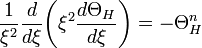$~\frac{1}{\xi^2} \frac{d}{d\xi}\biggl( \xi^2 \frac{d\Theta_H}{d\xi} \biggr) = - \Theta_H^n$
Known
Analytic
Solutions
via
Direct
Numerical
Integration
via
Self-Consistent
Field (SCF)
Technique

Free Energy
of
Bipolytropes

(nc, ne) = (5, 1)

### Stability Analysis

1D STABILITY

Pressure-Truncated
Isothermal
Spheres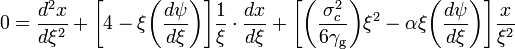$~0 = \frac{d^2x}{d\xi^2} + \biggl[4 - \xi \biggl( \frac{d\psi}{d\xi} \biggr) \biggr] \frac{1}{\xi} \cdot \frac{dx}{d\xi} + \biggl[ \biggl( \frac{\sigma_c^2}{6\gamma_\mathrm{g}}\biggr)\xi^2 - \alpha \xi \biggl( \frac{d\psi}{d\xi} \biggr) \biggr] \frac{x}{\xi^2}$ where: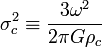$~\sigma_c^2 \equiv \frac{3\omega^2}{2\pi G\rho_c}$     and,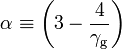$~\alpha \equiv \biggl(3 - \frac{4}{\gamma_\mathrm{g}}\biggr)$
via
Direct
Numerical
Integration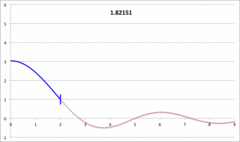Yabushita's
Analytic Sol'n
for
Marginally Unstable
Configurations
(1974)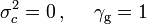$~\sigma_c^2 = 0 \, , ~~~~\gamma_\mathrm{g} = 1$ and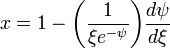$~x = 1 - \biggl( \frac{1}{\xi e^{-\psi}}\biggr) \frac{d\psi}{d\xi}$

Polytropes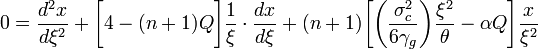$~0 = \frac{d^2x}{d\xi^2} + \biggl[ 4 - (n+1) Q \biggr] \frac{1}{\xi} \cdot \frac{dx}{d\xi} + (n+1) \biggl[ \biggl( \frac{\sigma_c^2}{6\gamma_g } \biggr) \frac{\xi^2}{\theta} - \alpha Q\biggr] \frac{x}{\xi^2}$ where: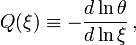$~Q(\xi) \equiv - \frac{d\ln\theta}{d\ln\xi} \, ,$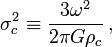$~\sigma_c^2 \equiv \frac{3\omega^2}{2\pi G\rho_c} \, ,$     and,$~\alpha \equiv \biggl(3 - \frac{4}{\gamma_\mathrm{g}}\biggr)$
Isolated
n = 3
Polytrope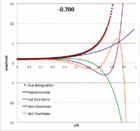Pressure-Truncated
n = 5
Configurations

Our Analytic Sol'n
for
Marginally Unstable
Configurations
(2017)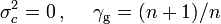$~\sigma_c^2 = 0 \, , ~~~~\gamma_\mathrm{g} = (n+1)/n$ and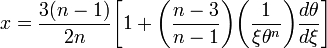$~x = \frac{3(n-1)}{2n}\biggl[1 + \biggl(\frac{n-3}{n-1}\biggr) \biggl( \frac{1}{\xi \theta^{n}}\biggr) \frac{d\theta}{d\xi}\biggr]$

1D DYNAMICS

## Two-Dimensional Configurations (Axisymmetric)

(Initially) Axisymmetric Configurations

### Axisymmetric Equilibrium Structures

2D STRUCTURE

#### Toroidal & Toroidal-Like

Definition: anchor ring

### Stability Analysis

2D STABILITY

#### Sheroidal & Spheroidal-Like

 The equilibrium models are calculated using the polytrope version (Bodenheimer & Ostriker 1973) of the Ostriker and Mark (1968) self-consistent field (SCF) code … the equilibrium models rotate on cylinders and are completely specified by$~n$, the total angular momentum, and the specific angular momentum distribution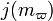$~j(m_\varpi)$. Here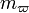$~m_\varpi$ is the mass contained within a cylinder of radius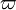$~\varpi$ centered on the rotation axis. The angular momentum distribution is prescribed in several ways: (1) imposing strict uniform rotation; (2) using the same$~j(m_\varpi)$ as that of a uniformly rotating spherical polybrope of index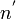$~n^'$ (see Bodenheimer and Ostriker 1973); and (3) using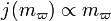$~j(m_\varpi) \propto m_\varpi$, which we refer to as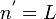$~n^' = L$,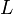$~L$ for "linear."

2D DYNAMICS

## Two-Dimensional Configurations (Nonaxisymmetric Disks)

Infinitesimally Thin, Nonaxisymmetric Disks

2D STRUCTURE

## Three-Dimensional Configurations

(Initially) Three-Dimensional Configurations

### Equilibrium Structures

3D STRUCTURE
 "One interesting aspect of our models … is the pulsation characteristic of the final central triaxial figure … our interest in the pulsations stems from a general concern about the equilibrium structure of self-gravitating, triaxial objects. In the past, attempts to construct hydrostatic models of any equilibrium, triaxial structure having both a high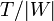$~T/|W|$ value and a compressible equation of state have met with very limited success … they have been thwarted by a lack of understanding of how to represent complex internal motions in a physically realistic way… We suggest … that a natural attribute of [such] configurations may be pulsation and that, as a result, a search for simple circulation hydrostatic analogs of such systems may prove to a fruitless endeavor. — Drawn from §IVa of Williams & Tohline (1988), ApJ, 334, 449
 Special numerical techniques must be developed "to build three-dimensional compressible equilibrium models with complicated flows." To date … "techniques have only been developed to build compressible equilibrium models of nonaxisymmetric configurations for a few systems with simplified rotational profiles, e.g., rigidly rotating systems (Hachisu & Eriguchi 1984; Hachisu 1986), irrotational systems (Uryū & Eriguchi 1998), and configurations that are stationary in the inertial frame (Uryū & Eriguchi 1996)." — Drawn from §1 of Ou (2006), ApJ, 639, 549

#### Binary Systems

• S. Chandrasekhar (1933), MNRAS, 93, 539: The equilibrium of distorted polytropes. IV. the rotational and the tidal distortions as functions of the density distribution
• S. Chandrasekhar (1963), ApJ, 138, 1182: The Equilibrium and the Stability of the Roche Ellipsoids
 Roche's problem is concerned with the equilibrium and the stability of rotating homogeneous masses which are, further, distorted by the constant tidal action of an attendant rigid spherical mass.

3D STABILITY

3D DYNAMICS

# See Also

 © 2014 - 2019 by Joel E. Tohline |   H_Book Home   |   YouTube   | Context: | PGE | SR | Appendices: | Equations | Variables | References | Binary Polytropes | Ramblings | Images | Images (2016 Layout) | ADS |